# Gauss Theorem

We know that there is always a static electric field around a positive or negative electrical charge and in that static electric field there is a flow of energy tube or flux. Actually this flux is radiated/emanated from the electric charge. Now amount of this flow of flux depends upon the quantity of charge it is emanating from. To find out this relation, the Gauss’s theorem was introduced. This theorem can be considered as one of the most powerful and most useful theorem in the field of electrical science. We can find out the amount of flux radiated through the surface area surrounding the charge from this theorem.This theorem states that the total electric flux through any closed surface surrounding a charge, is equal to the net positive charge enclosed by that surface.
Suppose the charges Q1, Q2_ _ _ _Qi, _ _ _ Qn are enclosed by a surface, then the theorem may be expressed mathematically by surface integral as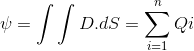Where, D is the flux density in coulombs/m2 and dS is the outwardly directed vector.

## Explanation of Gauss’s Theorem

For explaining the Gauss’s theorem, it is better to go through an example for proper understanding.
Let Q be the charge at the center of a sphere and the flux emanated from the charge is normal to the surface. Now, this theorem states that the total flux emanated from the charge will be equal to Q coulombs and this can be proved mathematically also. But what about when the charge is not placed at the center but at any point other than the center (as shown in the figure).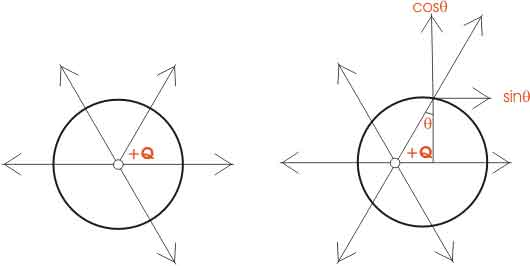At that time, the flux lines are not normal to the surface surrounding the charge, then this flux is resolved into two components which are perpendicular to each other, the horizontal one is the sinθ component and the vertical one is the cosθ component. Now when the sum of these components is taken for all the charges, then the net result is equal to the total charge of the system which proves Gauss’s theorem.

### Proof of Gauss’s Theorem

Let us consider a point charge Q located in a homogeneous isotropic medium of permittivity ε.The electric field intensity at any point at a distance r from the charge is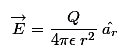The flux density is given as,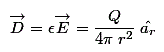Now from the figure the flux through area dS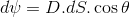Where, θ is the angle between D and the normal to dS.
Now, dScosθ is the projection of dS is normal to the radius vector. By definition of a solid angle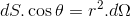Where, dΩ is the solid angle subtended at Q by the elementary surface are dS. So the total displacement of flux through the entire surface area is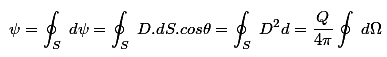Now, we know that the solid angle subtended by any closed surface is 4π steradians, so the total electric flux through the entire surface is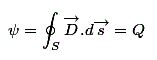This is the integral form of Gauss’s theorem. And hence this theorem is proved.

Want To Learn Faster? 🎓
Get electrical articles delivered to your inbox every week.
No credit card required—it’s 100% free.